Homework Help Question & Answers

# 1: Arrow and Apple Suppose that an apple of mass M is hanging motionless on a...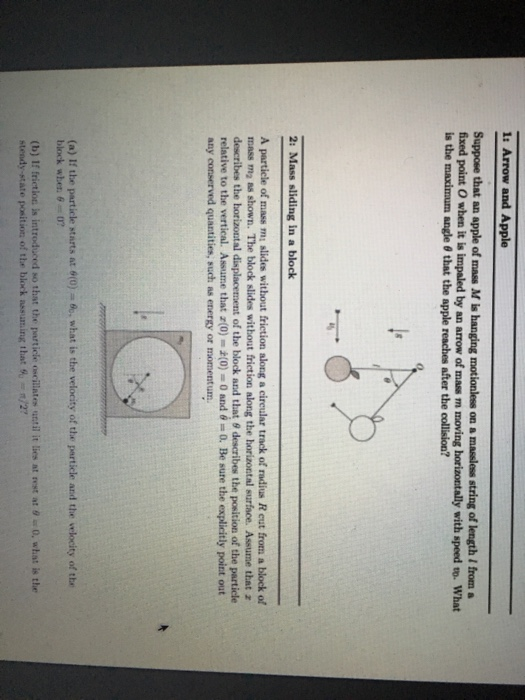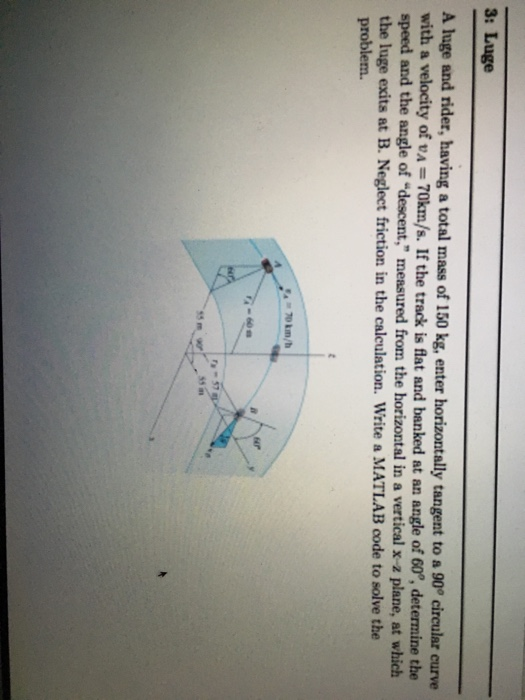1: Arrow and Apple Suppose that an apple of mass M is hanging motionless on a masless string of length / from a fixed point O when it is impaled by an arrow of mass m moving horizontally with speed to. What is the maximum angle θ that the apple reaches after the collision? 2: Mass sliding in a block A particle of mass m slides without friction along a cireular track of radius R eut from a block of mass ma ss shown. The block slides without friction along the horizontal surface. Assume that z describes te bratt al displacement of the block and that θ describes the position of the particle relative to the vertical. Assume that z(0)- (0-0 and θ = 0. Be sure the explicitly point out any conserved quantities, such as energy or momentum (a) If the particle starts at 0(0)ュ6," what is the velocity of the perticle and the velocity of the block when θ=0? (b) If frictioc is introduced so that the rarticle 'ncillate, until it lies nt at θ " 0, what is the

#### Homework Answers

Answer #1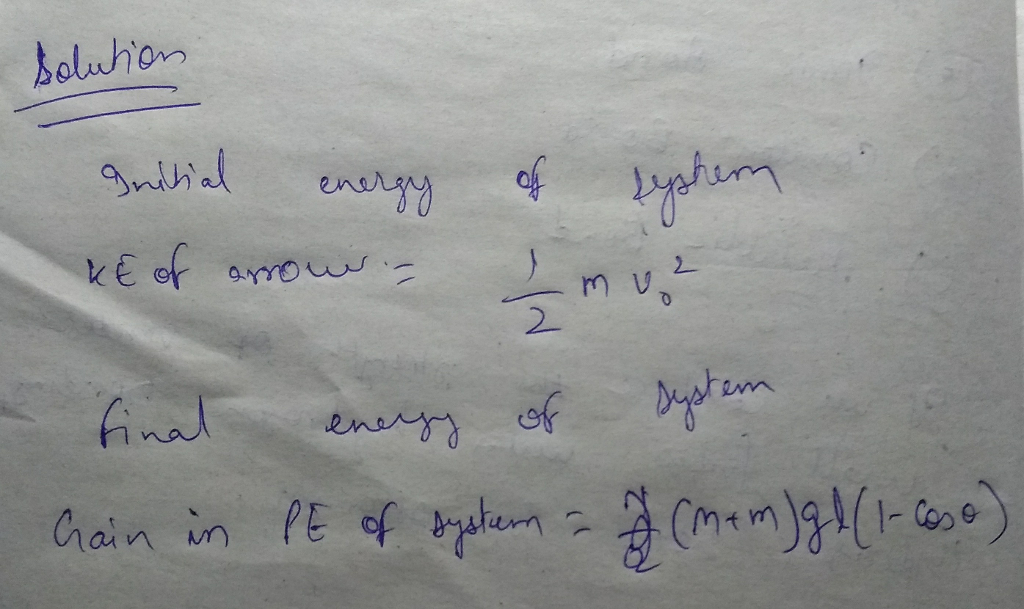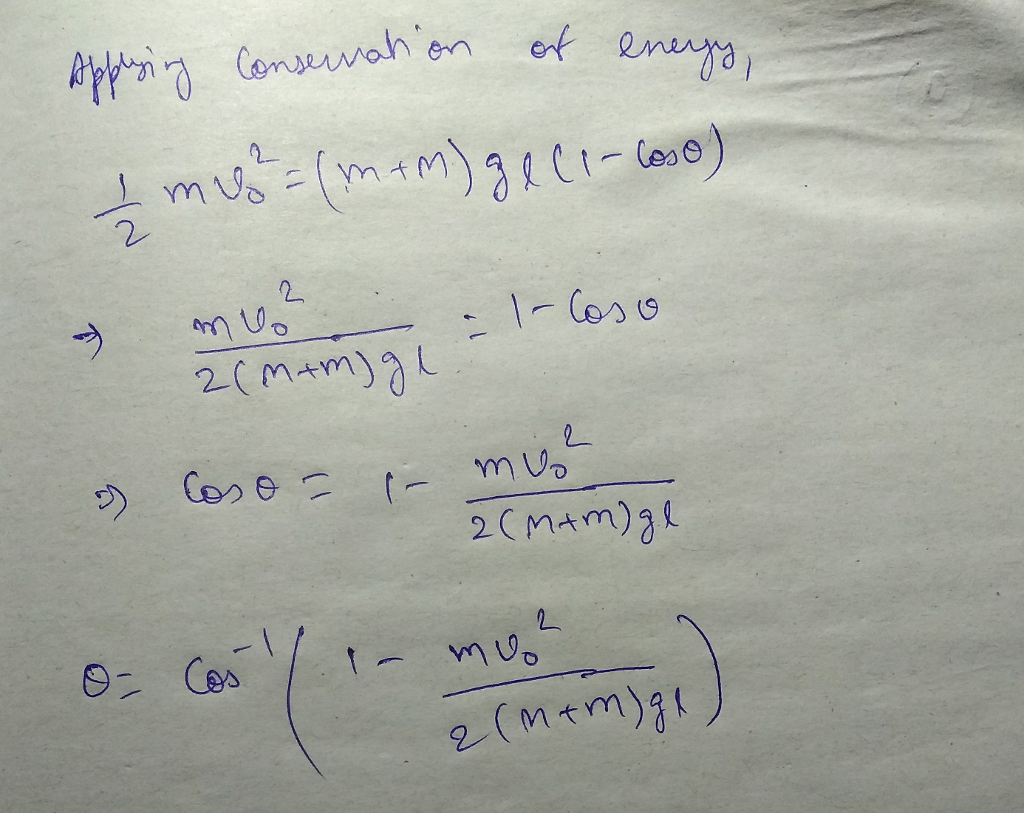Know the answer?
Your Answer:

#### Post as a guest

Your Name:

What's your source?

#### Earn Coin

Coins can be redeemed for fabulous gifts.

Not the answer you're looking for? Ask your own homework help question. Our experts will answer your question WITHIN MINUTES for Free.
Similar Homework Help Questions
• ### In the figure below, the hanging object has a mass of m, = 0.480 kg; the...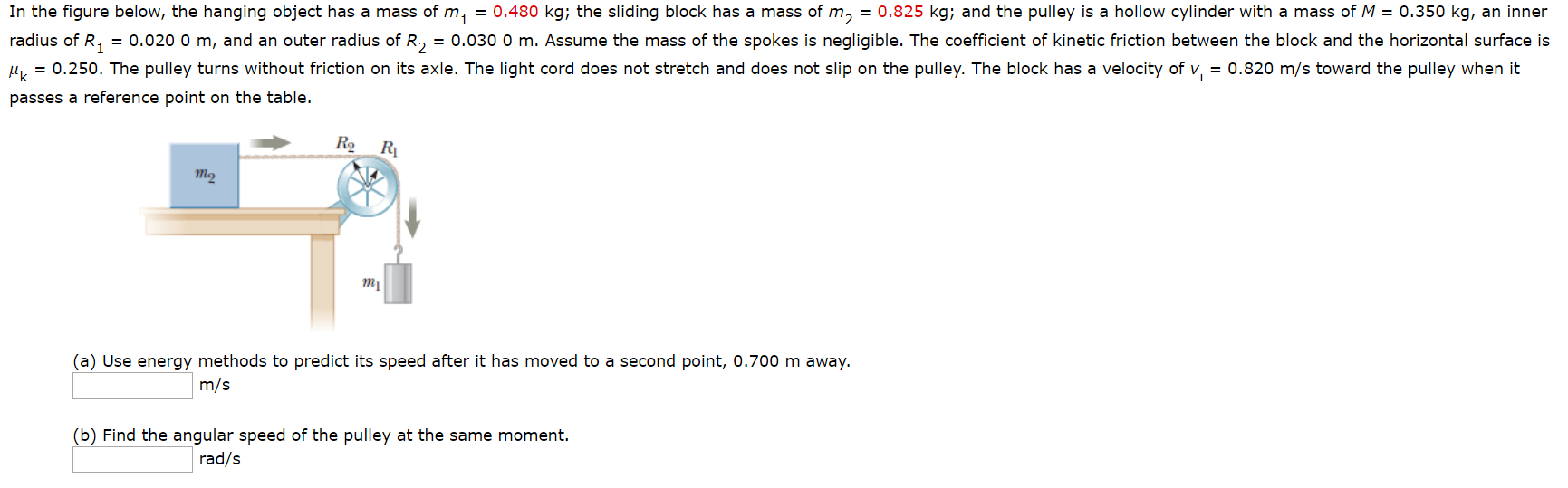In the figure below, the hanging object has a mass of m, = 0.480 kg; the sliding block has a mass of m, = 0.825 kg; and the pulley is a hollow cylinder with a mass of M = 0.350 kg, an inner radius of R4 = 0.020 0 m, and an outer radius of R, = 0.030 0 m. Assume the mass of the spokes is negligible. The coefficient of kinetic friction between the block and the horizontal surface...

• ### In the figure below, the hanging object has a mass of m_1 = 0.400 kg; the...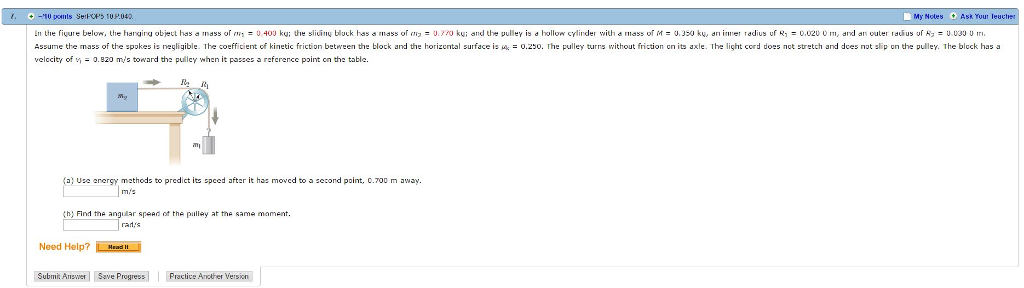In the figure below, the hanging object has a mass of m_1 = 0.400 kg; the sliding block has a mass of m_2 = 0.770 kg; and the pulley is a hollow cylinder with a mass of M = 0.350 kg, an inner radius of R_1 = 0.020 0 m, and an cuter radius of R_3 = 0.030 0 m. Assume the mass of the spokes is negligible. The coefficient of kinetic friction between the block and the horizontal surface...

• ### A Block of mass M

A block of mass M is held motionless on a frictionless inclined plane by means of a string attached to a vertical wall.If the string breaks, what is the magnitude of the acceleration of the block as it slides down the inclined plane?Assume the plane is not frictionless and the string breaks, what minimum value of the coefficient of static friction, us, would prevent the block from sliding down theinclined plane?

• ### In the figure below, the hanging object has a mass of m1 -0.480 kg; the sliding block has a mass ...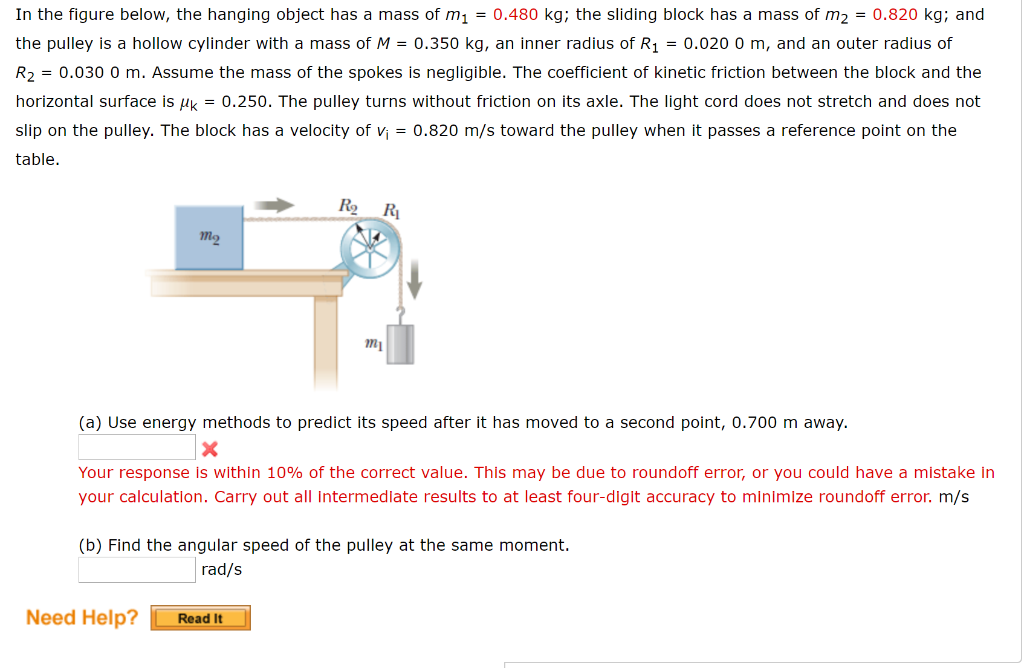In the figure below, the hanging object has a mass of m1 -0.480 kg; the sliding block has a mass of m2 0.820 kg; and the pulley is a hollow cylinder with a mass of M0.350 kg, an inner radius of R10.020 0 m, and an outer radius of R2 = 0.030 0 m. Assume the mass of the spokes is negligible. The coefficient of kinetic friction between the block and the horizontal surface is Hk0.250. The pulley turns without...

• ### A hanging weight, with a mass of me = 0.350 kg, is attached by a rope...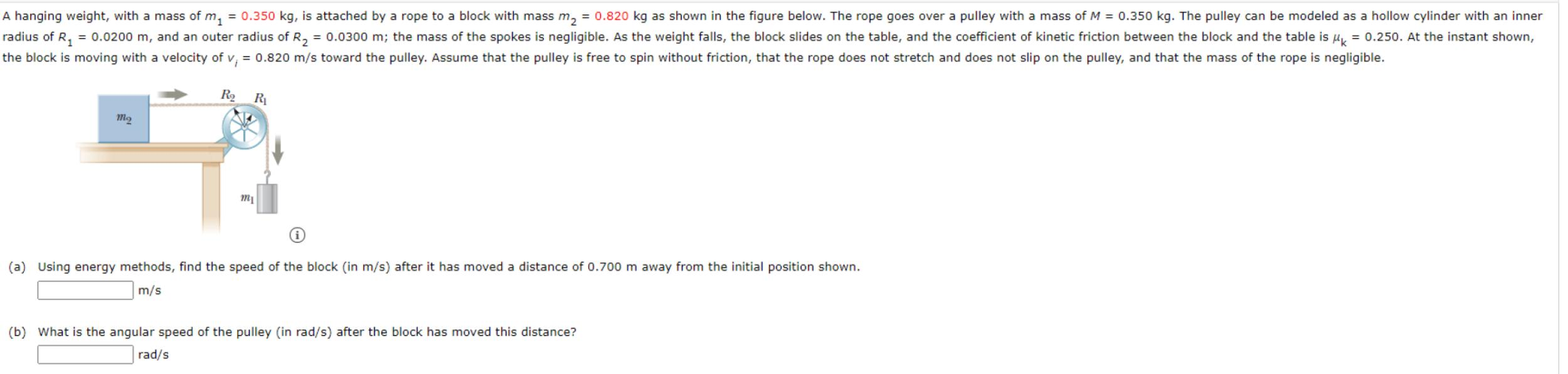A hanging weight, with a mass of me = 0.350 kg, is attached by a rope to a block with mass m2 = 0.820 kg as shown in the figure below. The rope goes over a pulley with a mass of M = 0.350 kg. The pulley can be modeled as a hollow cylinder with an inner radius of R2 = 0.0200 m, and an outer radius of R2 = 0.0300 m; the mass of the spokes is negligible. As...

• ### A hanging weight, with a mass of m1 = 0.365 kg, is attached by a rope...

A hanging weight, with a mass of m1 = 0.365 kg, is attached by a rope to a block with mass m2 = 0.825 kg as shown in the figure below. The rope goes over a pulley with a mass of M = 0.350 kg. The pulley can be modeled as a hollow cylinder with an inner radius of R1 = 0.0200 m, and an outer radius of R2 = 0.0300 m; the mass of the spokes is negligible. As...

• ### A hanging weight, with a mass of m, -0.370, is attached by a rope to a...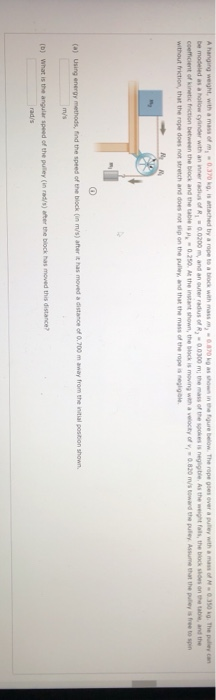A hanging weight, with a mass of m, -0.370, is attached by a rope to a block with mass-0070 Ahow in the gure below. The rope goes over a pulley with a f.350 G. The pulley can be modeled as a hollow cylinder with an inner radius of R = 0.0200, and an outer radius of R, -0.0300 m the mass of the spoke is negligible. As the weight fals, the black shoes on the table, and the coefficient of...

• ### 5. A particle of mass m slides without friction and starting from rest down a block...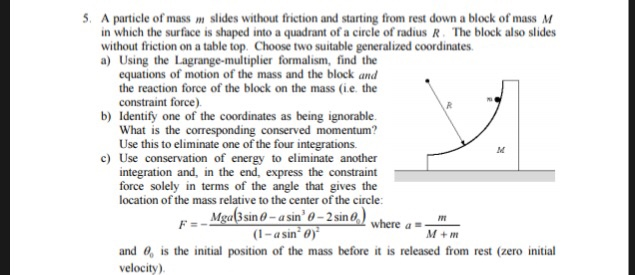5. A particle of mass m slides without friction and starting from rest down a block of mass M in which the surface is shaped into a quadrant of a circle of radius R. The block also slides without friction on a table top. Choose two suitable generalized coordinates a) Using the Lagrange-multiplier formalism, find the equations of motion of the mass and the block and the reaction force of the block on the mass (ie. the constraint force b)...

• ### A mass M slides upward along a rough plane surface inclined at angle \Theta\:Θ= 0.1 in...

A mass M slides upward along a rough plane surface inclined at angle \Theta\:Θ= 0.1 in radians to the horizontal. Initially the mass has a speed V_0\:V 0 = 3.42 m/s, before it slides a distance L = 1.0 m up the incline. After sliding this distance the new speed of the mass isV_0\:V 0 / 6 measured in m/s. What is the coefficient of kinetic friction \mu_kμ k between the mass and the incline?

• ### In the figure below, the hanging object has a mass of m1 -0.480 kg; the sliding...In the figure below, the hanging object has a mass of m1 -0.480 kg; the sliding block has a mass of m2 0.820 kg; and the pulley is a hollow cylinder with a mass of M0.350 kg, an inner radius of R10.020 0 m, and an outer radius of R2 = 0.030 0 m. Assume the mass of the spokes is negligible. The coefficient of kinetic friction between the block and the horizontal surface is Hk0.250. The pulley turns without...

Free Homework App

Scan Your Homework
to Get Instant Free Answers
Need Online Homework Help?

Get Answers For Free
Most questions answered within 3 hours.